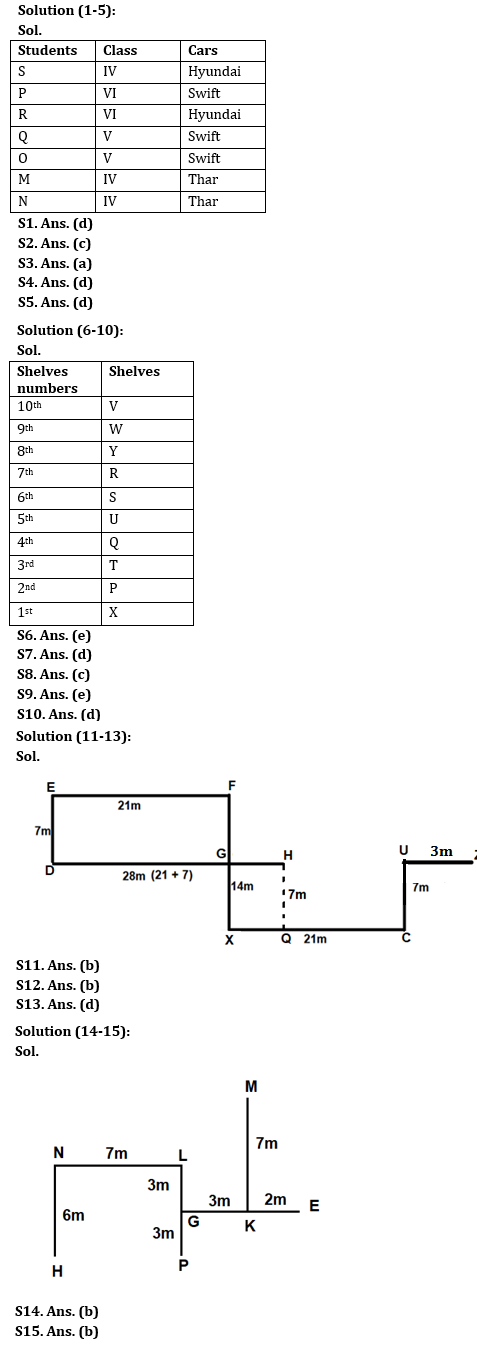Latest Banking jobs   »

# Reasoning Ability Quiz For IBPS RRB PO Clerk Prelims 2022- 12th August

Directions (1-5): Answer the questions based on the information given below.
Seven students M, N, O, P, Q, R and S study in three classes (IV, V and Vl) and they like three type of cars (Swift, Thar, Hyundai) such that at least two student study in the same class and at least two student like the same type of car.
Only Q studies in the same class as O and both of them like the same car. S study in class IV and likes Hyundai. Two students who study in class IV like Thar. P study in class Vl and likes Swift. Only R studies in the same class as P and likes Hyundai.

Q1. Who among the following student study in class IV?
(a) M
(b) N
(c) R
(d) Both M and N
(e) None of these

Q2. Who among the following student likes Swift?
(a) M
(b) N
(c) Q
(d) Either N or Q
(e) None of these

Q3. N studies in which of the following class?
(a) Class IV
(b) Class V
(c) Class VI
(d) Either IV or VI
(e) None of these

Q4. Which of the following combination of students represents the students who like Swift?
(a) P, M, O
(b) P, N, Q
(c) P, M, N
(d) Q, O, P
(e) None of these

Q5. Choose the incorrect combination of student – class – car from the given alternatives.
(a) Q – Class V – Swift
(b) M – Class IV – Thar
(c) N – Class IV – Thar
(d) M – Class IV – Hyundai
(e) O – Class V – Swift

Directions (6-10): Answer the questions based on the information given below.
There are 10 shelves (P, Q, R, S, T, U, V, W, X and Y) in a display cupboard but not necessarily in the same order. The bottommost shelf is number as one and the top most shelf is number as 10.
As many shelves are above P as below W which is not a prime number shelf. S is three places above T. Y is two places below V. Only three shelves are above R. Two shelves are between R and Q. U and Q are adjacent shelves. U is three places above P.

Q6. How many shelves are below shelf X?
(a) One
(b) Two
(c) Three
(d) Four
(e) None

Q7. Which of the following shelf is prime numbered shelf?
(a) U
(b) T
(c) Q
(d) Both U and T
(e) None of these

Q8. Which of the following shelf is three places below shelf Y?
(a) R
(b) W
(c) U
(d) Q
(e) None of these

Q9. S is which of the following shelf?
(a) 5th
(b) 9th
(c) 8th
(d) 10th
(e) None of these

Q10. How many shelves are between shelf T and shelf W?
(a) One
(b) Three
(c)Four
(d) Five
(e) None of these

Directions (11-13): Answer the questions based on the information given below.
Point U is 7m north of point C which is 21m east of point X. Point D is 7m south of point E which is 21m west of point of F. Point F is 14m north of point X. Point H is 28m east of point D. Point G is the midpoint between point F and point X. Point Z is in 3m east of point U.

Q11. If point Q is 7m south of point H then which of the following statement is true?
(a) Point Q is in west of point X
(b) Point Q is 14m away from point C
(c) Point Q is north-west of point F
(d) Point Q is south-west of point E
(e) None of the above

Q12. What is the direction of point Z with respect of X?
(a) North-west
(b) North-east
(c) South-west
(d) South-east
(e) None of the above

Q13. What is the direction of point C with respect of E?
(a) North-west
(b) North-east
(c) South-west
(d) South-east
(e) None of the above

Directions (14-15): Answer the questions based on the information given below.
Eight points (E, G, H, K, L, M, N, and P) are at different directions and distances with respect to one another. Point G is 3m west of Point K which is 7m south of Point M. Point E is 5m east of Point G which is 3m north of Point P. Point L is 3m north of Point G. Point L is 7m east of Point N which is 6m north of Point H.

Q14. The distance between point P and point H is same as the distance between point K and point _____.
(a) G
(b) M
(c) N
(d) L
(e) None of the above

Q15. What is the direction of point E with respect of point H?
(a) North-west
(b) North-east
(c) South-west
(d) South-east
(e) None of the above

Solutions#### Congratulations!Download Hindu Review of October 2021: Free PDF